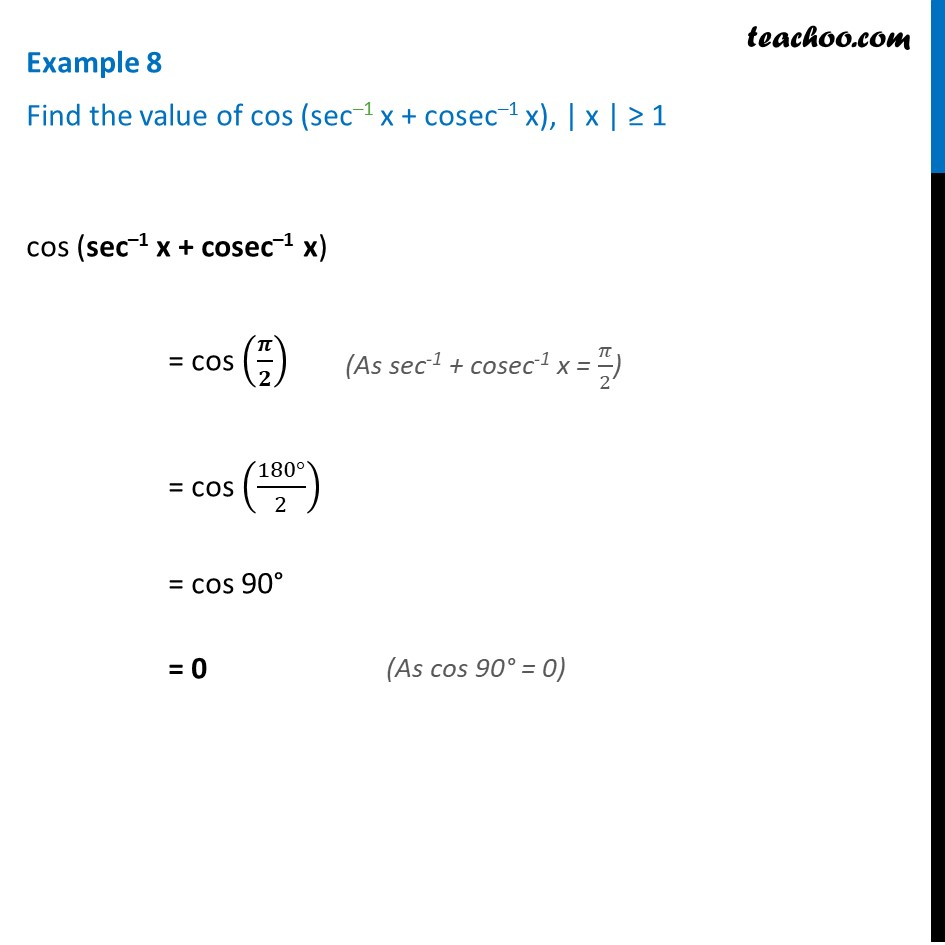1. Chapter 2 Class 12 Inverse Trigonometric Functions (Term 1)
2. Serial order wise
3. Examples

Transcript

Example 8 Find the value of cos (sec–1 x + cosec–1 x), | x | ≥ 1 cos (sec–1 x + cosec–1 x) = cos (𝝅/𝟐) = cos ((180°)/2) = cos 90° = 0 (As sec-1 + cosec-1 x = 𝜋/2)